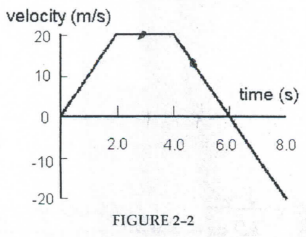# Problem: Figure 2.2 shows the velocity time graph of a motorist during a 8 second period. The motorist leaves home (position x = 0m) at time t = 0s and the velocity change as shown. What is the acceleration at 3.0 s?

###### FREE Expert Solution
84% (377 ratings)
###### Problem Details

Figure 2.2 shows the velocity time graph of a motorist during a 8 second period. The motorist leaves home (position x = 0m) at time t = 0s and the velocity change as shown. What is the acceleration at 3.0 s?Frequently Asked Questions

What scientific concept do you need to know in order to solve this problem?

Our tutors have indicated that to solve this problem you will need to apply the Velocity-Time Graphs & Acceleration concept. You can view video lessons to learn Velocity-Time Graphs & Acceleration. Or if you need more Velocity-Time Graphs & Acceleration practice, you can also practice Velocity-Time Graphs & Acceleration practice problems.

How long does this problem take to solve?

Our expert Physics tutor, Jeffery took 1 minute and 36 seconds to solve this problem. You can follow their steps in the video explanation above.

What professor is this problem relevant for?

Based on our data, we think this problem is relevant for Professor Graf's class at SUNY.